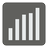Median
Elementary+
English EL ES FR HU JA KO PT-BR RU UK ZH-HANS
We have prepared a set of Editor's Choice Solutions. You will see them first after you solve the mission. In order to see all other solutions you should change the filter.A median is a numerical value separating the upper half of a sorted array of numbers from the lower half. In a list where there are an odd number of entities, the median is the number found in the middle of the array. If the array contains an even number of entities, then there is no single middle value, instead the median becomes the average of the two numbers found in the middle. For this mission, you are given a non-empty array of natural numbers (X). With it, you must separate the upper half of the numbers from the lower half and find the median.

Input: An array as a list of integers.

Output: The median as a float or an integer.

Example:

```checkio([1, 2, 3, 4, 5]) == 3
checkio([3, 1, 2, 5, 3]) == 3
checkio([1, 300, 2, 200, 1]) == 2
checkio([3, 6, 20, 99, 10, 15]) == 12.5
```

How it is used: The median has usage for Statistics and Probability theory, it has especially significant value for skewed distribution. For example: we want to know average wealth of people from a set of data -- 100 people earn \$100 in month and 10 people earn \$1,000,000. If we average it out, we get \$91,000. This is weird value and does nothing to show us the real picture. In this case the median would give to us more useful value and a better picture. The Article at Wikipedia.

Precondition:
1 < len(data) ≤ 1000
all(0 ≤ x < 10 ** 6 for x in data)

You should be an authorized user in order to see the full description and start solving this mission.